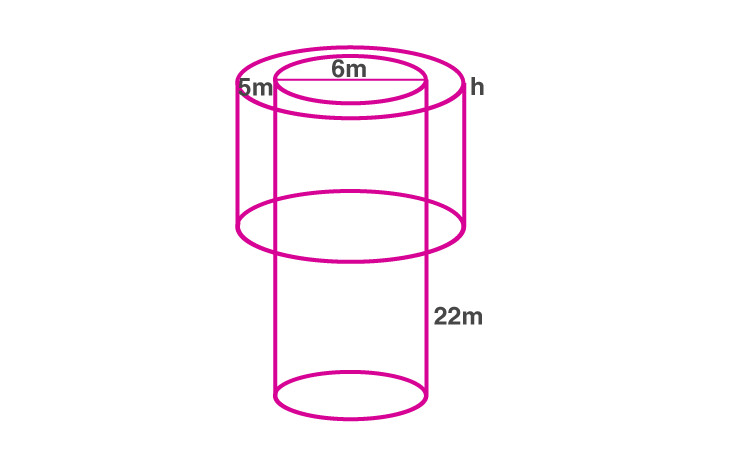Newbie

# A well with inner diameter 6 m is dug 22 m deep. Soil taken out of it has been spread evenly all round it to a width of 5 m to form an embankment. Find the height of the embankment.

• -1

An Important Question of class 10 Based on Mensuration Chapter of M.L Aggarwal for ICSE BOARD.
Here a well given with inner diameter 6 m is dug 22 m deep.
The Soil taken out of it has been spread evenly all round it to a width of 5 m to form an embankment.
Find the height of the embankment.
This is the Question Number 14, Exercise 17.5 of M.L Aggarwal.

Share

1.Given inner diameter of the well = 6 m

Radius of the well, r = 6/2 = 3 m

Depth of the well, H = 22 m

Volume of the soil dug out of well = r2H

= ×32×22

= 198 m3

Width of the embankment = 5 m

Inner radius of embankment, r = 3 m

Outer radius of embankment, R = 3+5 = 8 m

Let h be height of the soil embankment.

Volume of the soil embankment = (R2– r2)h

= (82– 32)h

= (64-9)h

= 55h

Volume of the soil dug out = volume of the soil embankment

198 = 55h

h = 198/55

h = 3.6 m

Hence the height of the soil embankment is 3.6 m.

• 1